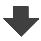Search:

# Chemical thermodynamics

## Calorimetry

### Exercise 2

Take the →   Table of specific thermal capacities by weight to determine the quantity of heat which must be added to $100\;g$ of copper to rise its temperature from $10^oC$ to $100^oC$.

$Q$ $=$ $c_{Cu}\cdot m\cdot (\theta_f$ $-$ $\theta_i)$ $=$ $400\cdot 0.1 \cdot (100$ $-$ $10)$ $=$ $3600\;J$